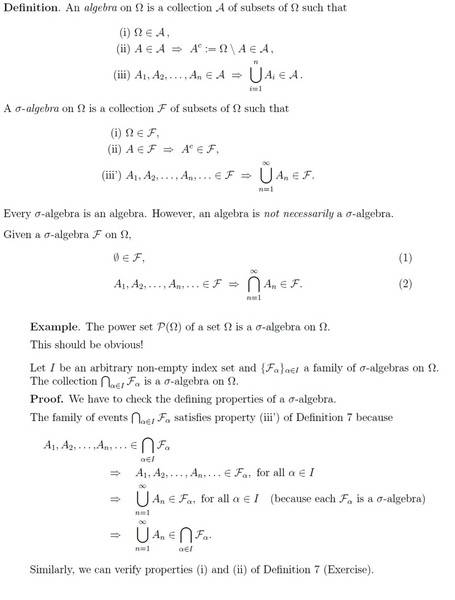# Sigma Algebra on Omega (Sample Space)

woundedtiger4Respected Members,

Suppose Ω is the set of eight possible outcomes of three coin tosses i.e. Ω={{HHH, HHT, HTH, HTTT, THH, THT, TTH, TTT}
So if we are not told the results then the sigma algebra ( denoted by F_α) at position α=0 is
F_0 = {∅, Ω}

Now if are told the first coin toss only then,
A_H={HHH, HHT, HTH, HTTT}, and A_T={THH, THT, TTH, TTT}
which the sigma Algebra at α=1 is
F_1={∅, Ω, A_H, A_T}

now in the attached picture the proof says that

A_1, A_2,....., A_n,.... ε ∩_αεI F

if we just consider two sigma algebras for our convenience to check this lets take the intersection of two above coin toss's sigma algebras i.e. F_0 and F_1

for I= 0 and 1,

∩_αεI F_α = F_0 ∩ F_1 = {∅, Ω} ∩ {∅, Ω, A_H, A_T} = {∅, Ω} ----(BETA)

the proof says that A_1, A_2,....., A_n,.... ε ∩_αεI F_α , and if we consider A_1 as A_H and A_2 as A_T then why are they not in the intersection of F_0 ∩ F_1 as shown in (BETA) ?

## Answers and Replies

economicsnerd
You have $\mathcal F_0=\{\emptyset,\Omega\}$ and $\mathcal F_1=\{\emptyset,A_H,A_T\Omega\}$. In particular, since $\mathcal F_0\subseteq \mathcal F_1$, your example has $\mathcal F_0\cap\mathcal F_1=\mathcal F_0$.

What you need for your hypothesis is $A_i \in \mathcal F_0\cap\mathcal F_1=\mathcal F_0=\{\emptyset,\Omega\}$ for every $i$. That is, every $A_i$ is either empty or the whole state space; in particular, you can't use $A_i=A_H$ or $A_i=A_T$. To verify the union, notice that the union is just $\Omega$ if at least one of your $A_i=\Omega$ and $\emptyset$ if (the only other possibility) every $A_i=\emptyset$. In particular, either way, $\bigcup_i A_i \in \mathcal F_0.$

woundedtiger4
Why not A_i=A_H or A_T ? Lets say, A_1={ø}, A_2={Omega}, A_3=A_H and A_4=A_T then A_1, A_2, A_3, A_4 belongs to intersection_alpha belongs to I F_alpha, here F_alpha is F_0
After all A_H and A_T are the subsets of Omega, and definition 7 says that algebra is a collection of subsets of Omega.

Ps. Sorry, I am on train and typing this message on mobile therefore I am unable to add accurate symbols :(